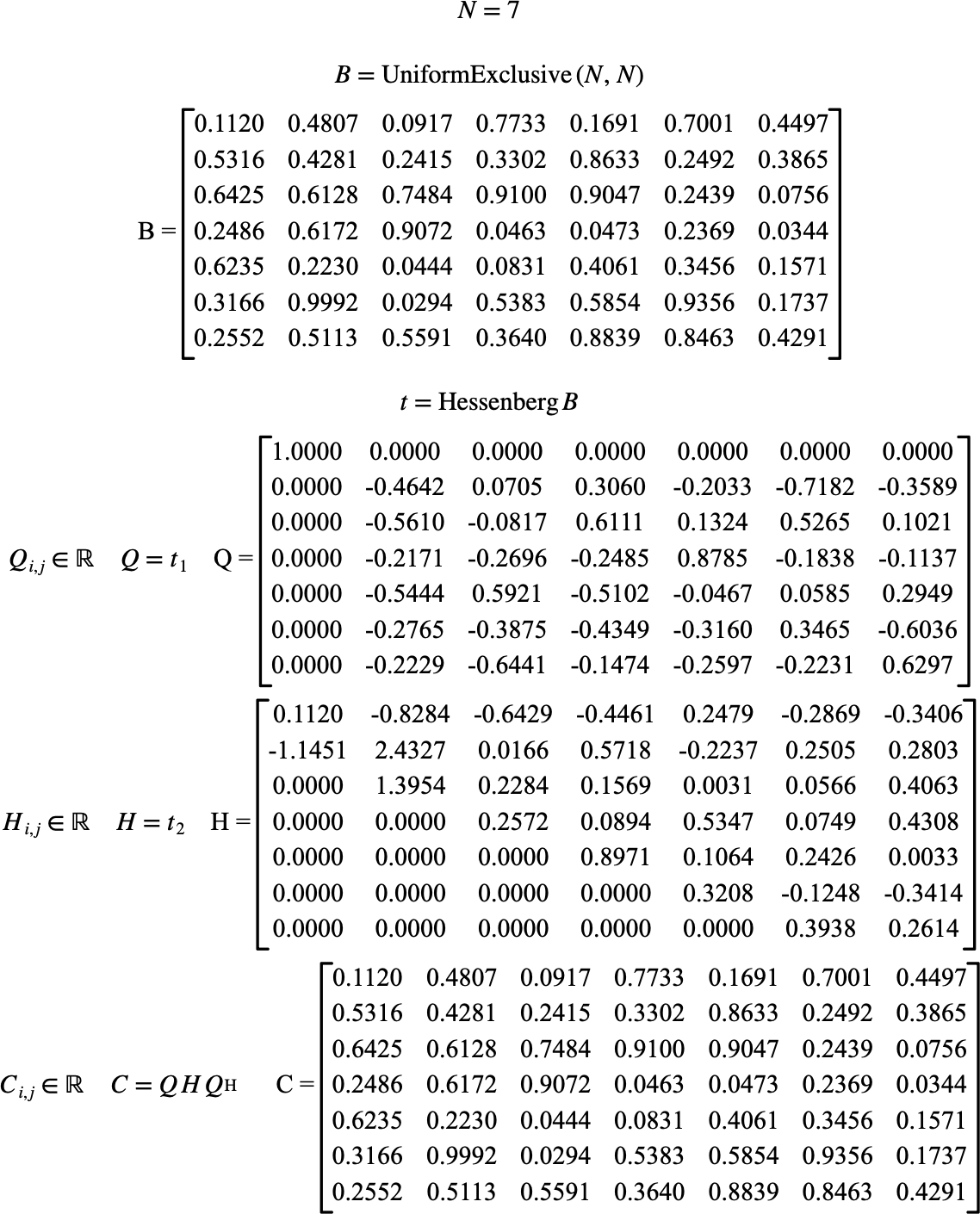# $$\text{Hessenberg}$$¶

You can use the $$\text{Hessenberg}$$ function to perform a Hessenberg decomposition of a real or complex matrix, creating $$Q H Q ^ T$$ or $$Q H Q^H$$ respectively.

You can use the \hessenberg backslash command to insert this function.

The following variants of this function are available:

• $$\text{tuple } \text{Hessenberg} \left ( \text{<matrix>} \right )$$

The $$\text{Hessenberg}$$ function is implemented for real and complex matrices although the function will convert boolean and integer matrices to a real matrix, if needed.

The $$\text{Hessenberg}$$ returns a tuple containing:

• The $$Q$$ unitary matrix, and

• The upper Hessenberg matrix, $$H$$.

Figure 148 shows the basic use of the $$\text{Hessenberg}$$ function.Figure 148 Example Use Of The Hessenberg Function